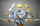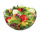# Fraction calculator

The calculator performs basic and advanced operations with fractions, expressions with fractions combined with integers, decimals, and mixed numbers. It also shows detailed step-by-step information about the fraction calculation procedure. Solve problems with two, three, or more fractions and numbers in one expression.

## Result:

### 5/15 = 1/3 ≅ 0.3333333

Spelled result in words is one third.

#### Rules for expressions with fractions:

Fractions - simply use a forward slash between the numerator and denominator, i.e., for five-hundredths, enter 5/100. If you are using mixed numbers, be sure to leave a single space between the whole and fraction part.
The slash separates the numerator (number above a fraction line) and denominator (number below).

Mixed numerals (mixed fractions or mixed numbers) write as integer separated by one space and fraction i.e., 1 2/3 (having the same sign). An example of a negative mixed fraction: -5 1/2.
Because slash is both signs for fraction line and division, we recommended use colon (:) as the operator of division fractions i.e., 1/2 : 3.

Decimals (decimal numbers) enter with a decimal point . and they are automatically converted to fractions - i.e. 1.45.

The colon : and slash / is the symbol of division. Can be used to divide mixed numbers 1 2/3 : 4 3/8 or can be used for write complex fractions i.e. 1/2 : 1/3.
An asterisk * or × is the symbol for multiplication.
Plus + is addition, minus sign - is subtraction and ()[] is mathematical parentheses.
The exponentiation/power symbol is ^ - for example: (7/8-4/5)^2 = (7/8-4/5)2

#### Examples:

subtracting fractions: 2/3 - 1/2
multiplying fractions: 7/8 * 3/9
dividing Fractions: 1/2 : 3/4
exponentiation of fraction: 3/5^3
fractional exponents: 16 ^ 1/2
adding fractions and mixed numbers: 8/5 + 6 2/7
dividing integer and fraction: 5 ÷ 1/2
complex fractions: 5/8 : 2 2/3
decimal to fraction: 0.625
Fraction to Decimal: 1/4
Fraction to Percent: 1/8 %
comparing fractions: 1/4 2/3
multiplying a fraction by a whole number: 6 * 3/4
square root of a fraction: sqrt(1/16)
reducing or simplifying the fraction (simplification) - dividing the numerator and denominator of a fraction by the same non-zero number - equivalent fraction: 4/22
expression with brackets: 1/3 * (1/2 - 3 3/8)
compound fraction: 3/4 of 5/7
fractions multiple: 2/3 of 3/5
divide to find the quotient: 3/5 ÷ 2/3

The calculator follows well-known rules for order of operations. The most common mnemonics for remembering this order of operations are:
PEMDAS - Parentheses, Exponents, Multiplication, Division, Addition, Subtraction.
BEDMAS - Brackets, Exponents, Division, Multiplication, Addition, Subtraction
BODMAS - Brackets, Of or Order, Division, Multiplication, Addition, Subtraction.
GEMDAS - Grouping Symbols - brackets (){}, Exponents, Multiplication, Division, Addition, Subtraction.
Be careful, always do multiplication and division before addition and subtraction. Some operators (+ and -) and (* and /) has the same priority and then must evaluate from left to right.

## Fractions in word problems:

• Divide 13Divide. Simplify your answer and write as an improper fraction or whole number. 14÷8/3
• Frac equation v23.80 is two fifths of a quarter sum of money. How much money is there?Max is working out 2/3+7/9. He says the answer is 9/12. What mistake have Max made?
• A quotientWhat is the quotient of 3/10 divided by 2/4 as a fraction?
• Fraction of a fractionWhat is two-quarters of twelve?
• Equation with xSolve the following equation: 2x- (8x + 1) - (x + 2) / 5 = 9
• Berry SmoothieRory has 5/8 cup of milk. How much milk does she have left after she doubles the recipe of the smoothie? Berry Smoothie: 2 cups strawberries 1 cup blueberries 1/4 cup milk 1 tbsp (tablespoon) sugar 1/2 tsp (teaspoon) lemon juice 1/8 tsp (teaspoon) vanilla
• MidnightHow many hours are, if the time that elapsed since 8:00 is 2/5 of the time that will past till midnight?We need two tenths kg of a carrot, one tenth of peas and three tenths of of tomatoes to make salad. Express the fraction of the weight of the vegetables to be salad. Convert the result to grams.The ratio of the number of giraffes to the number of monkeys in a zoo is 2 to 5. Which statement about the giraffes and monkeys could be true? A. For every 10 monkeys in the zoo, there are 4 giraffes. B. For every  giraffe in the zoo, there are 3 monkeys.Find the unknown number equal to a quarter of a fifth of a number, which is by 152 more than an unknown number.Subtract mixed number with regrouping: 11 17/20- 6 19/20Jacobs mom bought two whole pizzas. He ate 2/10 of the pizza and his dad ate 1 1/5. How much is left.